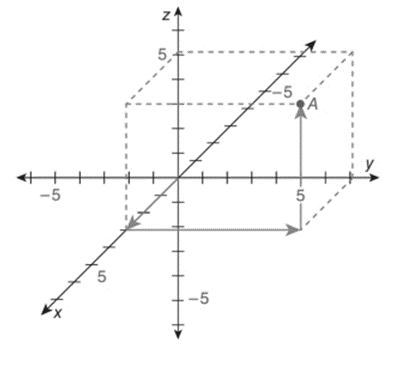Chapter 10.6, Problem 1E### Elementary Geometry for College St...

6th Edition
Daniel C. Alexander + 1 other
ISBN: 9781285195698

#### Solutions

Chapter
Section### Elementary Geometry for College St...

6th Edition
Daniel C. Alexander + 1 other
ISBN: 9781285195698
Textbook Problem
1 views

# In the Cartesian coordinate system below, name the ordered triple x ,   y ,   z represented by point A. Also, plot the point B 5 ,   6 ,   4 .To determine

To name:

The ordered triple found in the given figure.

Explanation

The given figure is shown below.

In a three dimensional Cartesian coordinate system, x-axis moves forward and backward.

Forward means positive coordinate.

Backward means negative coordinate.

y-axis moves right and left.

Right means positive coordinate.

Left means negative coordinate.

z-axis moves upward and downward.

Upward means positive coordinate.

Downward means negative coordinate.

In the given figure,

Beginning from the origin, x-axis moves 3 units forward, y-axis moves 7 units to theright and z-axis moves 5 units upwards to arrive at the given point A.

Hence, the ordered triple is A3, 7, 5

### Still sussing out bartleby?

Check out a sample textbook solution.

See a sample solution

#### The Solution to Your Study Problems

Bartleby provides explanations to thousands of textbook problems written by our experts, many with advanced degrees!

Get Started

#### Which of the following three graphs is the graph of ? Explain your choice.

Mathematical Applications for the Management, Life, and Social Sciences

#### Convert the expressions in Exercises 6584 to power form. x3

Finite Mathematics and Applied Calculus (MindTap Course List)

#### 2,339+118+3,650+8,770+81+6=

Contemporary Mathematics for Business & Consumers